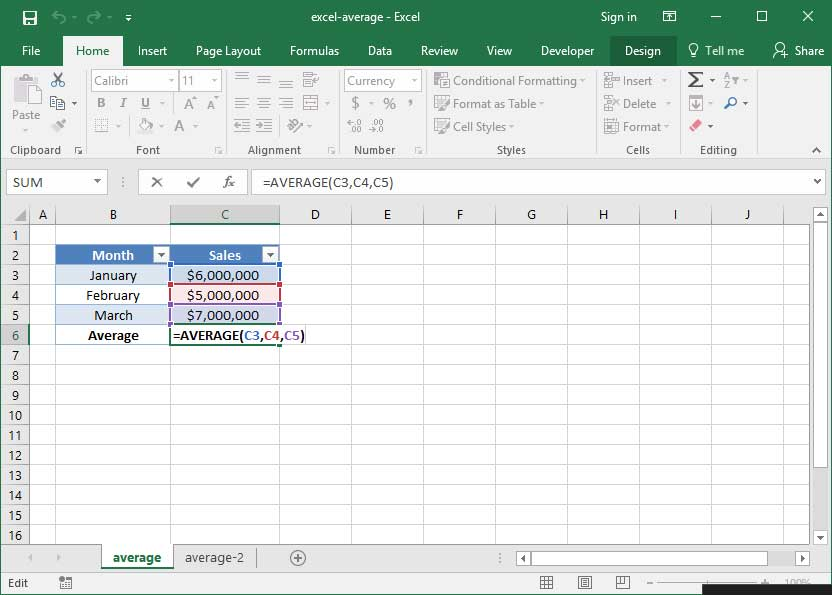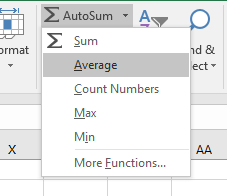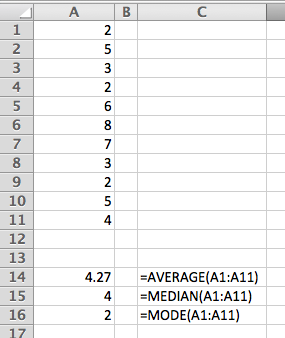• # What is the average height of students in a class?

• What is the average monthly electricity bill during a year?
• And What is the average number of days that employees get sick? By men? By women?

Of course, there are situations that are useless. for example:

80% of drivers consider themselves better than average!

## 3 methods of calculating the average value

You may remember from school math lessons that there are three types of averages: median, mode, and mean.

• To work with MEDIAN or Excel, Excel sorts the values ​​of your data in order (behind the scenes), and then selects the middle value.
• To prepare a MODE, Excel sorts the values ​​of your data in order (behind the scenes), then selects the most repeated value.
• And To calculate the MEAN or average, Excel collects the total values ​​of your data (behind the scenes), then divides the value by the number of data values ​​in the list.

## How to calculate MEAN or average in Excel

The simplest and most common way to calculate the average value for a set of numbers is to use the AVERAGE function in Excel. This function uses the MEAN method, which collects the data and then divides the answer by the number of values.

AVERAGE function structure:

= AVERAGE (number1, number2…)

for example:

= AVERAGE (A1, A2, A3)

Or

= AVERAGE (A1: A11)

This function includes all the items in the brackets and then divides them by their number. It can be selected from the AutoSum drop-down menu or by entering it directly into the cell.

If you have values ​​in cells A1 through A11, follow these steps to use the AutoSum tool to calculate the mean:

Select 11 cells.

2. Click the AutoSum icon on the slider.

3. Select AVERAGE from the list.Or if you want to write the formula manually:

1. Select an empty cell that contains the calculated mean.

2. Type AVERAGE =. (You can not use AVG or any abbreviated word of AVERAGE)

3. Select cells A1 to A11 with your mouse.

4- Type the symbol). (Closed bracket)

5. Press Enter.

You have to use every technique to get results.

= AVERAGE (A1: A11)

## How to calculate the MEDIAN or middle value in Excel

The second method is to use the Excel MEDIAN function. This function views the sorted sequence of data (behind the scenes) and then selects the middle value.

MEDIAN function structure:

= MEDIAN (number1, number 2…)

for example:

= MEDIAN (A1: A11)

## How to calculate the value of MODE or mode in Excel

The third and last method is to use the Excel MODE function. This function views the sorted sequence of data (behind the scenes), then selects the data with the most repetition.

MODE function structure:

= MODE (number1, number 2…)

for example:

= MODE (A1: A11)

## Conclusion

Finally, how to compare 3 different functions is given: